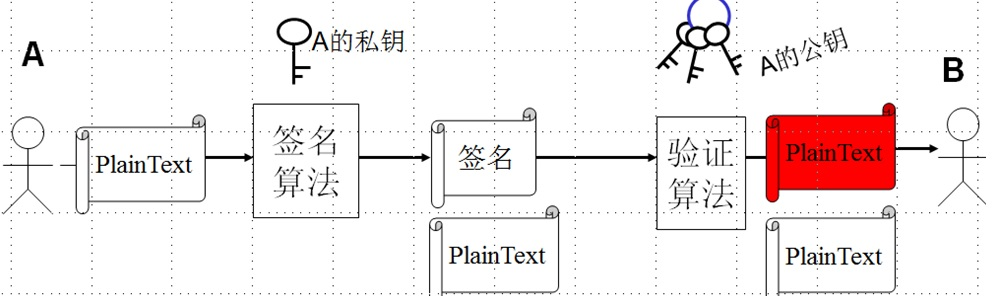RSA数字签名算法演示程序实现 (python) 100C1、RSA算法实现可以直接调用编程语言提供的接口函数实现，不需要自行编写密码算法；
2、RSA密钥对的数据可以从文件读取和存储
3、发送方和接收方的数据传输方面，可以是C/S模式，也可以是B/S模式进行传输；（需要网络编程）
4、界面设计要求：
1）RSA密钥对简单生成过程的图形化演示，密钥对可以用文件形式保存和读取
2）采用图形化在界面中完整的演示RSA的数字签名过程（如图所示），发方的签名消息可以采用文件形式保存进行，接收方可以读取文件获得签名消息和公钥。
3）接收方和发送方数据传输采用网络编程实现

3个回答

100C币就找人做项目啊，这把程序员当成要饭的了。你花费100C币就是为了黑程序员？

``````
``````RSA 公私匙 数字签名
RSA中通过KeyPair来生成公私匙 ``` KeyPairGenerator keyPairGen = KeyPairGenerator.getInstance("RSA"); keyPairGen.initialize(1024); KeyPair keyPair = keyPairGen.generateKeyPair(); RSAPublicKey publicKey = (RSAPublicKey) keyPair.getPublic(); RSAPrivateKey privateKey = (RSAPrivateKey) keyPair.getPrivate(); ``` 在做数字签名或者加解密时，为什么不直接使用上门的公私匙 而是要对公私匙做转化 比如公匙 X509EncodedKeySpec keySpec = new X509EncodedKeySpec(publicKey.getEncoded()); KeyFactory keyFactory = KeyFactory.getInstance(KEY_ALGORITHM); PublicKey publicK = keyFactory.generatePublic(keySpec); ``` 比如私匙 PKCS8EncodedKeySpec pkcs8KeySpec = new PKCS8EncodedKeySpec(privateKey.getEncoded()); KeyFactory keyFactory = KeyFactory.getInstance(KEY_ALGORITHM); PrivateKey privateK = keyFactory.generatePrivate(pkcs8KeySpec); 目前网上的帖子基本都是用转化后的公私匙 但自己在方法里试了下 在签名 验签方法里如果直接使用 不转化的 也是可以做数字签名的，那这几步转化的意义何在，求达人告知下 ，谢谢！
RSA算法在python中的实现总是无法正确算出信息，请大神帮看下
# 先贴个源代码，代码我是从网络上找的，他是能用的。我在想好像是python不支持32位以上的运算？所以在解码的时候溢出 ``` # -*- coding: cp936 -*- # FileName:RSA Encode # encode formula:(message**e) mod n = result # uncode formula:(result**d) mod n = message # prime key=d; public key=e,n import random import numpy as np import os ######################################################## def encode(message,e=1,n=2): result=np.mod(message**e,n) return result ######################################################### def decode(result=5,key=1,n=2): print '密文：',result,'密钥：',key,'公钥：',n try1=result**key print 'message**key=',try1 message=np.mod(try1,n) return message ######################################################### def localset(pmin=3,pmax=10): plistcheck=[] # 在取值范围内的非质数列表 plist=[] # 在取值范围内的质数列表 local=[] # 获取一定范围内的质数 for p1 in range(pmin,pmax): for i in range(2,p1/2): if np.mod(p1,i)==0: plistcheck=plistcheck+[p1] break for each in range(pmin,pmax): if each in plistcheck: pass else: plist=plist+[each] print plist ######################################################### # 随机获取公钥n p1=random.choice(plist) p2=random.choice(plist) print 'p1=',p1 print 'p2=',p2 n=p1*p2 f=(p1-1)*(p2-1) local=local+[n] print 'n=',n ######################################################### # 设定一个公钥e e=3 local=local+[e] print 'e=',e ######################################################### # 计算密钥d k=0 while np.mod((k*f+1),e)!=0: k=k+1 if k>20000000: print 'k=',k print '出错！' os._exit(0) d=(k*f+1)/e local=local+[d] print 'd=',d return local ############################################################## def main(message=89,pmin=5,pmax=15): local=localset(pmin,pmax) message=input('请输入你希望加密的信息：') Encrypted=encode(message,local,local) messagecheck=decode(Encrypted,local,local) print 'locallist=',local print 'message=',message print 'result=',Encrypted print 'messagecheck=',messagecheck ############################################################### #暂定加密信息为 pmin=input('请输入最小值：') pmax=input('请输入最大值：') main(pmin=pmin,pmax=pmax) ```

C#实现RSA签名，签名算法采用SHA-256，JAVA部分已有，因需要转为C#代码，望大神出手相助
package test; import java.math.BigInteger; import java.security.KeyFactory; import java.security.MessageDigest; import java.security.PrivateKey; import java.security.Signature; import java.security.spec.RSAPrivateKeySpec; import java.util.Calendar; import java.util.Date; public class test { public static void main(String[] args) throws Exception { String msg = "0|20140411|1\n银联基金交易账号|用户在基金公司的账号|申请日期|银联订单号|确认日期|基金公司确认编号|返回码|失败原因\n1040000000371674|20000015|20140410|20120528990000120140410000000120|20140411|2250000000016|0000|\n"; byte[] data = msg.getBytes("UTF-8"); // 私钥 BigInteger modulus = new BigInteger("00af9133ade333df8b9aabd776334d0d8f5d96597fe2041fff716185305934cc7a386ab451f28156730ce8a76adcf9bf93ffc87fa47c0d60c4652f1700f50304997a20563cc854f1f84c921f9b1f72d0d3e989d4b22669014859135e89ced24bbac3af1d1a4a76ff5736f81fdc461d2f96cebc5f36eacae7981e7ea59443448fa1", 16); BigInteger privateExponent = new BigInteger("009c46140920e6c881bc405f7e1ff5e32f0cafcaa4912af8cc6840d4cc0f6b76e3079abb3d022dec246686249773707efd9ebcfa19102edc46948650e49232888116e4b54fe77b6eaf5061db4f50c89646241559f602d477efb0d6b755a67a40e978753f56d16142660c47bc91408cf01deaaf3f30180050e2730980e9498cdd3d", 16); RSAPrivateKeySpec privateKeySpec = new RSAPrivateKeySpec(modulus, privateExponent); KeyFactory keyFactory = KeyFactory.getInstance("RSA"); PrivateKey privateKey = keyFactory.generatePrivate(privateKeySpec); //用SHA-256做摘要 MessageDigest md= MessageDigest.getInstance("SHA-256"); data=md.digest(data); // 签名 Signature instance = Signature.getInstance("SHA1withRSA"); instance.initSign(privateKey); instance.update(data); byte[] sing = instance.sign(); System.out.println(byteArr2HexString(sing)); } // 以下为调用到的公共方法 public static String byteArr2HexString(byte[] bytearr) { if (bytearr == null) { return "null"; } StringBuffer sb = new StringBuffer(); for (int k = 0; k < bytearr.length; k++) { if ((bytearr[k] & 0xFF) < 16) { sb.append("0"); } sb.append(Integer.toString(bytearr[k] & 0xFF, 16)); } return sb.toString(); } }
1024位rsa算法c++实现

RSA加密算法中对于相同的数，分别用Key1和Key2进行加密，加密的结果与密钥的顺序是无关的吗?
python import rsa 运行报错

RSA 加密算法的计算
Problem Description RSA is one of the most powerful methods to encrypt data. The RSA algorithm is described as follow: > choose two large prime integer p, q > calculate n = p × q, calculate F(n) = (p - 1) × (q - 1) > choose an integer e(1 < e < F(n)), making gcd(e, F(n)) = 1, e will be the public key > calculate d, making d × e mod F(n) = 1 mod F(n), and d will be the private key You can encrypt data with this method : C = E(m) = me mod n When you want to decrypt data, use this method : M = D(c) = cd mod n Here, c is an integer ASCII value of a letter of cryptograph and m is an integer ASCII value of a letter of plain text. Now given p, q, e and some cryptograph, your task is to "translate" the cryptograph into plain text. Input Each case will begin with four integers p, q, e, l followed by a line of cryptograph. The integers p, q, e, l will be in the range of 32-bit integer. The cryptograph consists of l integers separated by blanks. Output For each case, output the plain text in a single line. You may assume that the correct result of plain text are visual ASCII letters, you should output them as visualable letters with no blank between them. Sample Input 101 103 7 11 7716 7746 7497 126 8486 4708 7746 623 7298 7357 3239 Sample Output I-LOVE-ACM.

RSA算法如何生成一个长度较短的秘钥

rsa的公钥和私钥生成过程我已经了解了, 现在生成了 公钥为 （n，e) = (4757 , 101)，私钥为 （n，d) = (4757 ，1601) 然后比如我要加密的数据是123 公钥加密123^101%4757=992 私钥解密992^1601%4757=123 上面的过程应该没有问题 我的问题是 0.公钥是可以完全公开的吗, 如果可以公开, 那要是别人知道密文了, 不就可以for i循环遍历明文, i^101%4757==992 来猜明文是多少了么 1.如何使用该算法加解密字符串的数据呢 请教各位前辈!!!谢谢了!!!!

JAVA里的rsa公钥加密128位的明文加出来的结果一定是512位的密文吗？可以加出来256位的密文么？
Java学习的正确打开方式

linux系列之常用运维命令整理笔录

Python十大装B语法
Python 是一种代表简单思想的语言，其语法相对简单，很容易上手。不过，如果就此小视 Python 语法的精妙和深邃，那就大错特错了。本文精心筛选了最能展现 Python 语法之精妙的十个知识点，并附上详细的实例代码。如能在实战中融会贯通、灵活使用，必将使代码更为精炼、高效，同时也会极大提升代码B格，使之看上去更老练，读起来更优雅。

2019年11月中国大陆编程语言排行榜
2019年11月2日，我统计了某招聘网站，获得有效程序员招聘数据9万条。针对招聘信息，提取编程语言关键字，并统计如下： 编程语言比例 rank pl_ percentage 1 java 33.62% 2 cpp 16.42% 3 c_sharp 12.82% 4 javascript 12.31% 5 python 7.93% 6 go 7.25% 7 p...

《奇巧淫技》系列-python！！每天早上八点自动发送天气预报邮件到QQ邮箱

SQL-小白最佳入门sql查询一

redis分布式锁，面试官请随便问，我都会

Nginx 原理和架构
Nginx 是一个免费的，开源的，高性能的 HTTP 服务器和反向代理，以及 IMAP / POP3 代理服务器。Nginx 以其高性能，稳定性，丰富的功能，简单的配置和低资源消耗而闻名。 Nginx 的整体架构 Nginx 里有一个 master 进程和多个 worker 进程。master 进程并不处理网络请求，主要负责调度工作进程：加载配置、启动工作进程及非停升级。worker 进程负责处...
Python 编程开发 实用经验和技巧
Python是一门很灵活的语言，也有很多实用的方法，有时候实现一个功能可以用多种方法实现，我这里总结了一些常用的方法和技巧，包括小数保留指定位小数、判断变量的数据类型、类方法@classmethod、制表符中文对齐、遍历字典、datetime.timedelta的使用等，会持续更新......
Idon’t know what that dream is that you have, I don't care how disappointing it might have been as you've been working toward that dream,but that dream that you’re holding in your mind, that it’s po...
“狗屁不通文章生成器”登顶GitHub热榜，分分钟写出万字形式主义大作

《程序人生》系列-这个程序员只用了20行代码就拿了冠军

11月8日，由中国信息通信研究院、中国通信标准化协会、中国互联网协会、可信区块链推进计划联合主办，科技行者协办的2019可信区块链峰会将在北京悠唐皇冠假日酒店开幕。 　　区块链技术被认为是继蒸汽机、电力、互联网之后，下一代颠覆性的核心技术。如果说蒸汽机释放了人类的生产力，电力解决了人类基本的生活需求，互联网彻底改变了信息传递的方式，区块链作为构造信任的技术有重要的价值。 　　1...
Java世界最常用的工具类库
Apache Commons Apache Commons有很多子项目 Google Guava 参考博客